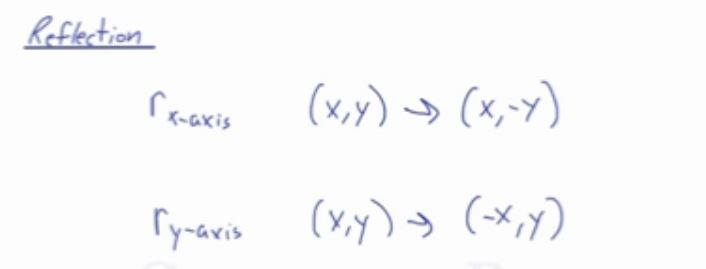In this video, you will learn how to do a reflection over an axis, such as the x-axis or y-axis.

To reflect a shape over an axis, you can either match the distance of a point to the axis on the other side of using the reflection notation.

To match the distance, you can count the number of units to the axis and plot a point on the corresponding point over the axis.

You can also negate the value depending on the line of reflection where the x-value is negated if the reflection is over the y-axis and the y-value is negated if the reflection is over the x-axis.

Either way, the answer is the same thing.

For example:
Triangle ABC with coordinate points A(1,2), B(3,5), and C(7,1). Determine the coordinate points of the image after a reflection over the x-axis.

Since the reflection applied is going to be over the x-axis, that means negating the y-value. As a result, points of the image are going to be:
A'(1,-2), B'(3,-5), and C'(7,-1)

By counting the units, we know that point A is located two units above the x-axis. Count two units below the x-axis and there is point A’. Do the same for the other points and the points are also
A'(1,-2), B'(3,-5), and C'(7,-1)

Reflection Notation:
rx-axis = (x,-y)
ry-axis = (-x,y)Video-Lesson Transcript

In this lesson, we’ll go over reflections on a coordinate system. This will involve changing the coordinates.

For example, try to reflect over the$x$-axis.

We have triangle$ABC$ with coordinates$A (1, 2)$$B (3, 5)$$C (7, 1)$

We’re going to reflect it over the$x$-axis. We’re going to flip it over.

So we’ll do what we normally do. Just one point at a time.

Now,$A$ is above$2$ units from the$x$-axis so we’ll move it below the$x$-axis by$2$ units.

This will be the$A^\prime (1, -2)$.

Let’s do the same for$B$. It’s$5$ units above the$x$-axis so we’re going to go$5$ units below the$x$-axis. Notice that it’s still in line with$x = 3$.

This is now$B^\prime (3, -5)$.

Look at point$C$ at$7, 1$. It’s$1$ point above the$x$-axis so we’ll go$1$ point below the$x$-axis.

So,$C^\prime (7, -1)$.

And just connect the points. Then we can see our reflection over the$x$-axis.

When we reflect over the$x$-axis, something happens to the coordinates.

The initial coordinates$(x, y)$ change. The$x$ coordinate stays the same but the$y$ coordinate is the same number but now it’s negative.$(x, y) \rightarrow (x, -y)$

In reflecting over the$x$-axis, we’ll write$r_{x-axis}$

Now, the same thing goes for reflecting over the$y$-axis.

We’re going to reflect triangle$ABC$ over the$y$-axis.$r_{y-axis} \triangle{ABC}$

Similar to reflecting over the$x$-axis, we’ll just do one point at a time.$A (1, 2)$$B (3, 5)$$C (7, 1)$$A$ is$1$ unit from the$y$-axis so we’ll move$1$ beyond the$y$-axis.

So,$A^\prime (-1, 2)$.

Let’s look at$B$ at$(3, 5)$. That means it’s$3$ units from the$y$-axis so we’ll move$3$ coordinates on the other side of the$y$-axis.

Now,$B^\prime (-3, 5)$.

Finally,$C$ is at$(7, 1)$ so we’ll go$7$ points beyond the$y$-axis.

We’ll have$C^\prime (-7, 1)$.

Now, we can draw a triangle that is a reflection of triangle$ABC$ over the$y$-axis.

Let’s look at how these coordinates changed.

Originally we have coordinates$(x, y)$ but$x$ became negative while$y$ stayed the same.$(x, y) \rightarrow (-x, y)$

Let’s recap.

The rule of reflecting over the$x$-axis is$r_{x-axis} (x, y) \rightarrow (x, -y)$

And for reflecting over the$y$-axis is$r_{y-axis} (x, y) \rightarrow (-x, y)$

If you reflect it over the$x$-axis,$x$ coordinate stays the same the other coordinate becomes negative.

And reflecting over the$y$-axis,$y$ coordinate stays the same while the other coordinate becomes negative.